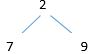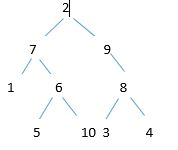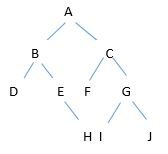# Post-order:

Post-order traverse need to traverse left, then right then root. Array, Linked List, Queues, Stacks, etc. are linear traversal where have only one logical way to traverse them. Though trees can be traversed in different ways. That Traversals:

1.  In-order (Left, Root, Right)
2. Preorder (Root, Left, Right)
3. Postorder (Left, Right, Root)

For Post-order traverse need to traverse left, then right then root.

Following figure Post -Order traverse 2, 7, 9.tree

## Algorithm for Post-order traverse.

Input tree, root.

Step 1: Traverse left sub tree like call Pre-order (tree, root. left).

Step 2: Traverse right sub tree like call Pre-order (tree, root. right).

Step 3: visit root node.

Now, Traverse the following tree by following program.tree

Traverse : 1, 5, 10, 6, 7, 3, 4, 8, 9, 2.

Recent Articles on Binary Tree in the blog   Topic :

1. Introduction Of Binary Tree
2. Traversals such as

## Implementation Process for Post-order traverse:

• 1st need to create node struct where data, *left , *right declared;
• 2nd need to create new node function which take an item and return new node, where firstly need i) memory allocation same as datatype size. If failed to memory allocation show error or assign data into new node,if left or right child no value then put them as NULL.
• 3rd  to add child left and right need two function both take two input one for node pointer another for left or right child assigned.
• 4th create create_tree() function by create_node ,add_left_child() and right_child() function. This function return root.
• 5th Now use post_order() recursion function, if left child not null call post_order() recursively for left node,Again if right child not null  call post_order()recursively for right node, root  print.
• 6th main() function receive root data or value will print.

## Implementation Using C :

A

#include <stdio.h>

typedef struct node Node;

struct node

{

int data;

Node  *left;

Node  *right;

};

Node *create_node (int item)

{

Node *new_node = (Node *) malloc(sizeof(Node));

if(new_node == NULL)

{

printf(“Error ! Could not create an Error\n”);

exit(1);

}

new_node -> data = item;

new_node -> left= NULL;

new_node ->right =NULL;

return (new_node);

}

void add_left_child( Node *node, Node *child )

{

node->left =child;

}

void add_right_child( Node *nodee, Node *child )

{

nodee->right =child;

}

Node *create_tree()

{

Node *two = create_node(2);

Node *seven=create_node(7);

Node *nine=create_node(9);

Node *one = create_node(1);

Node *six = create_node(6);

Node *five=create_node(5) ;

Node *ten = create_node(10);

Node *eight = create_node(8);

Node *three=create_node(3);

Node *four=create_node(4);

return two;

}

void post_order(Node *node)

{

if(node->left != NULL)

{

post_order(node->left);

}

if(node->right != NULL)

{

post_order(node->right);

}

printf(“%d “,node->data);

}

struct Node node;

int main()

{

Node *root =create_tree();

post_order(root);

printf(” “);

return 0;

}

OUTPUT: 1 5 10 6 7 3 4 8 9 2

##### Data Structure more articles## In-order Traversal of a Tree.

In-order traversal is very commonly used on binary search trees(BST).Tt returns values from the underlying set in order, according to the comparator set up of the binary search tree. Array, Linked List, Queues, Stacks, etc. are linear traversal where have only one logical way to traverse them. Though trees can be traversed in different ways. That Traversals:

1.  In-order (Left, Root, Right)
2. Preorder (Root, Left, Right)
3. Postorder (Left, Right, Root)

## In-order:

For In-order traverse need to traverse left, then root, then right.tree

Following figure In-order traverse  7,2,9.

## Algorithm for In-order traverse.

Input tree, root.

Step 1: Traverse left sub tree like call In-order (tree, root. left).

Step 2: visit root node.

Step 3: Traverse right sub tree like call In-order(tree, root. right).

Now, Traverse the following tree by following program.tree

Traverse : 1,7,5,6,10,2,9,3,8,4.

Recent Articles on Binary Tree in the blog   Topic :

1. Introduction Of Binary Tree
2. Traversals such as

## Implementation Process:

1st need to create node struct where data, *left , *right declared;

2nd need to create new node function which take an item and return new node, where firstly need i) memory allocation same as datatype size. If failed to memory allocation show error or assign data into new node, if left or right child no value then put them as NULL.

3rd  to add child left and right need two function both take two input one for node pointer another for left or right child assigned.

4th create create_tree() function by create_node ,add_left_child() and right_child() function. This function return root.

5th Now use in_order() recursion function, if left child not null call in_order() recursively for left node. root  print , then Again if right child not null  call in_order()recursively for right node.

6th main() function receive root data or value will print.

## Program:

#include <stdio.h>

typedef struct node Node;

/* A binary tree node has data, pointer to left child and a pointer to right child */

struct node

{

int data;

Node  *left;

Node  *right;

};

Node *create_node (int item)

{

Node *new_node = (Node *) malloc(sizeof(Node)); /* create storage*/

if(new_node == NULL) { printf(“Error ! Could not create an Error\n”);

exit(1);

}

new_node -> data = item;

new_node -> left= NULL;

new_node ->right =NULL;

return (new_node);

}

void add_left_child( Node *node, Node *child )

{

node->left =child;

}

void add_right_child( Node *nodee, Node *child )

{

nodee->right =child;

}

Node *create_tree()

{

Node *two = create_node(2);

Node *seven=create_node(7);

Node *nine=create_node(9);

Node *one = create_node(1);

Node *six = create_node(6);

Node *five=create_node(5) ;

Node *ten = create_node(10);

Node *eight = create_node(8);

Node *three=create_node(3);

Node *four=create_node(4);

return two;

}

/* print tree nodes according to the in order traversal. */

void in_order(Node *node)

{

if(node->left != NULL)

{

in_order(node->left);

}

printf(“%d\n”,node->data);

if(node->right != NULL)

{

in_order(node->right);

}

}

//struct Node node; int main()

{

/* Given a binary tree, to print its nodes according to the in order traversal. */

Node *root =create_tree();

in_order(root);

printf(“\n”);

return 0;

}

## Data Structure more articles

1. PostOrder traverse C Program For Pre_Order traversal in a Tree.
2. In-order traverse C program In_order Traversal of a Tree.
3. Post order traversal Post-order traverse of a Tree with C program.## Pre-order traversal:

Pre-order traverse need to traverse root, then left, then right. Array, Linked List, Queues, Stacks, etc. are linear traversal where have only one logical way to traverse them. Though trees can be traversed in different ways. That Traversals:

1.  In-order (Left, Root, Right)
2. Preorder (Root, Left, Right)
3. Postorder (Left, Right, Root)

For Pre-order traverse need to traverse root, then left, then right.tree

Following figure Pre–order traverse  2, 7, 9.

• Input tree, root.
• Step 1: visit root node.
• Step 2: Traverse left sub tree like call Pre-order (tree, root. left).
• Step 3: Traverse right sub tree like call Pre-order (tree, root. right).

Now, Traverse the following tree by following program.tree

Traverse : 2,7,1,6,5,10,9,8,3,4.

Recent Articles on Binary Tree in the blog   Topic :

1. Introduction Of Binary Tree
2. Traversals such as

## C Implemetation Process:

• 1st need to create node struct where data, *left , *right declared;
• 2nd need to create new node function which take an item and return new node, where firstly need i) memory allocation same as datatype size.

If failed to memory allocation show error or assign data into new node,if left or right child no value then put them as NULL.

• 3rd  to add child left and right need two function both take two input one for node pointer another for left or right child assigned.
• 4th create create_tree() function by create_node ,add_left_child() and right_child() function. This function return root.
• 5th Now use pre_order() recursion function, root  print first, then if left child not null call pre_order() recursively for left node. Again if right child not null  call pre_order()recursively for right node.
• 6th main() function receive root data or value will print.

C Program For Pre-order traversal in a Tree.

#include <stdio.h>

/*create a data type node */

typedef struct node Node;

struct node{

int data;

Node  *left;

Node  *right;

};

/*Create each node and corresponding child.Allocate memory for new node if new node empty. show error either keep item data variable,initialize left and right child into NULL then return new */

Node *create_node (int item) {

Node *new_node = (Node *) malloc(sizeof(Node));

if(new_node == NULL)

{

printf(“Error ! Could not create an tree\n”);

exit(1);

}

new_node -> data = item;

new_node -> left= NULL;

new_node ->right =NULL;

return (new_node);

}

/*add left node corresponding parent node */

void add_left_child( Node *node, Node *child ) {

node->left =child;

}

/*add right node corresponding parent node */

void add_right_child( Node *node, Node *child ) {

node->right =child;

}

/*create tree for node (2,7),(2,9),(7,1),(7,6),(6,5),(6,10),(9,8),(8,3),(8,4)*/

Node *create_tree() {

Node *two = create_node(2);/*create root node: 2 */

Node *seven=create_node(7);/*create root node: 7 */

Node *nine=create_node(9);/*create root node: 9 */

Node *one = create_node(1); /*create parent node 1 */

Node *six = create_node(6);/*create parent node:6 */

Node *five=create_node(5) ;/* similar process rest of all*/

Node *three=create_node(3);

Node *four=create_node(4);

return two;/* return root node*/

} /*print root node 2; */

void pre_order(Node *node){

printf(“%d\n”,node->data);

if(node->left != NULL){

pre-order(node->left);}

else if(node->right != NULL){

pre-order(node->right);}}

int main() {

Node *root =create_tree();

pre_order(root);

/*printf(“%d”,root->data);*/

printf(” “);

return 0;

}

Data Structure more articles

1. PostOrder traverse C Program For Pre_Order traversal in a Tree.
2. In order traverse C program In_order Traversal of a Tree.
3. Post order traversal Post-order traverse of a Tree with C program.# Binary Tree:

A binary tree each node has at most two children generally referred as left child and right child from root node.

Components of Each node:

• Pointer to left subtree
• Pointer to right subtree
• Data element

The topmost node in the tree is called the root. An empty tree is represented by NULL pointer.

A tree which has two nodes corresponding their parent node is known as binary tree.

Binary tree term as two types.

1)      Full Binary tree.

A tree which has two nodes corresponding their parent node is known as full binary tree.

2)      Complete Binary tree.

3)      A tree which has left nodes corresponding their parent node is known as complete binary tree.### Common Terminologies of Binary Tree:

• Root: Topmost node in a tree.
• Parent: Every node (excluding a root) in a tree is connected by a directed edge from exactly one other node. This node is called a parent.
• Child: A node directly connected to another node when moving away from the root.
• Leaf/External node: Node with no children.
• Internal node: Node with atleast one children.
• Depth of a node: Number of edges from root to the node.
• Height of a node: Number of edges from the node to the deepest leaf. Height of the tree is the height of the root.Binary Tree

In the  binary tree root node is A. The tree has 10 nodes with 5 internal nodes A,B,C,E,G and 5 external nodes D,F,H,I,J. The height of the tree is 3. B is the parent of D and E while D and E are children of B.

## C implementation Process:

1st need to create node struct where data, *left , *right declared;

2nd need to create new node function which take an item and return new node, where firstly need i) memory allocation same as datatype size.

If failed to memory allocation show error or assign data into new node,if left or right child no value then put them as NULL.

3rd  to add child left and right need two function both take two input one for node pointer another for left or right child assigned.

4th create create_tree() function by create_node ,add_left_child() and right_child() function. This function return root.

5th main() function receive root data or value will print.

### C Program For binary tree.

#include <stdio.h>
/*create a data type node */
typedef struct node Node;
struct node{
int data;
Node  *left;
Node  *right;
};

/*Create each node and corresponding child.Allocate memory for new node if new node empty. show error either keep item data variable,initialize left and right child into NULL then return new */

Node *create_node (int item)

{
Node *new_node = (Node *) malloc(sizeof(Node));
if(new_node == NULL)
{
printf(“Error ! Could not create an tree\n”);
exit(1);
}
new_node -> data = item;
new_node -> left= NULL;
new_node ->right =NULL;
return (new_node);
}
/*add left node corresponding parent node */

void add_left_child( Node *node, Node *child )

{
node->left =child;
}
/*add right node corresponding parent node */
void add_right_child( Node *node, Node *child )

{
node->right =child;
}Node *seven=create_node(7);/*create root node: 7 */
Node *nine=create_node(9);/*create root node: 9 */
Node *one = create_node(1); /*create parent node 1 */
Node *six = create_node(6);/*create parent node:6 */
Node *five=create_node(5) ;/* similar process rest of all*/
Node *three=create_node(3);
Node *four=create_node(4);
return two;/* return root node*/
} /*print root node 2; */

int main()

{

Node *root =create_tree();

printf(“%d”,root->data);

return 0;

}

Recent Articles on Binary Tree in the blog   Topic :

1. Introduction Of Binary Tree
2. Traversals such as